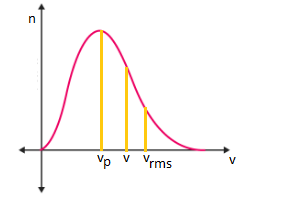# Important Kinetic Theory of Gas Formulas For JEE Main and Advanced

## Kinetic Theory of Gas Formulas

1. Boltzmann’s constant

kB=nR/N

kB is the Boltzmann’s constant

R is the gas constant

n is the number of moles

N is the number of particles in one mole (the Avogadro number)

2. Total translational K.E of gas

K.E= (3/2)nRT

n is the number of moles

R is the universal gas constant

T is the absolute temperature

3. Maxwell distribution lawVrms ＞ V＞ Vp

Vrms is the RMS speed

V is the Average speed

Vp is the most probable speed

4. RMS speed (Vrms)

$V_{rms}=\sqrt{\frac{3kt}{m}}=\sqrt{\frac{3RT}{M}}$

R is the universal gas constant

T is the absolute temperature

M is the molar mass

5. Average Speed (V)

$\vec{v}=\sqrt{\frac{8kt}{\pi m}}=\sqrt{\frac{8RT}{\pi M}}$

6. Most probable speed (Vp)

$v_{p}=\sqrt{\frac{2kt}{m}}=\sqrt{\frac{2RT}{M}}$

7. Pressure of ideal gas

p=⅓ ρ v2rms

ρ is the density of molecules

8. Equipartition of energy

K=½ kBT for each degree of freedom

K=(f/2) kBT for molecules having f degrees of freedom

kB is the Boltzmann’s constant

T is the temperature of the gas

9. Internal energy

U = (f/2)nRT

For n moles of an ideal gas.

Test your knowledge on Important Kinetic Theory Of Gas Formulas For Jee